# Intermediate Algebra Tutorial 37

Intermediate AlgebraAnswer/Discussion to 1a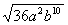The thought behind this is that we are looking for the square root of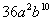. This means that we are looking for an expression that, when we square it, we getWhat do you think it is?

Let's find out if you are right: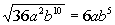Since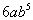squared is,is the square root ofAnswer/Discussion to 1b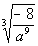The thought behind this is that we are looking for the cube root of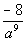. This means that we are looking for an expression that, when we cube it, we get.

What do you think it is?

Let's find out if you are right:Since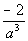cubed isis the cube root ofAnswer/Discussion to 1c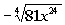The thought behind this is that we are looking for the fourth root of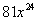. This means that we are looking for an expression that, when we raise it to the fourth power,  we get.

What do you think it is?

Let's find out if you are right: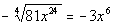Since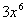raised to the fourth power isand there is a negative in front of the radical,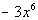is the negative fourth root ofAnswer/Discussion to 1d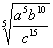The thought behind this is that we are looking for the fifth root of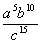.  This means that we are looking for an expression that, when we raise it to the fifth power, we getWhat do you think it is?

Let's find out if you are right: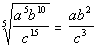Since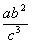raised to the fifth power  isis the fifth root of.Answer/Discussion to 2a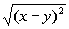Since it didn't say that x or y are positive, we have to assume that they can be either positive or negative.  Since the root number and exponent are equal, then we can use the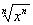rule.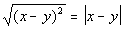Since the root number and the exponent inside are equal and are the even number 2, then we need to put an absolute value around x - y for our answer.  The reason for the absolute value is that we do not know if x or y are positive or negative.  So if we put x - y as our answer and it was negative, it would not be a true statement.Answer/Discussion to 2b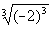Since the root number and exponent are equal then we can use therule.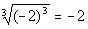This time our root number and exponent were both the odd number 3.  When an odd numbered root and exponent match, then the answer is the base whether it is negative or positive.